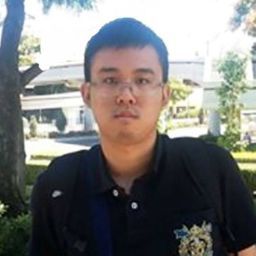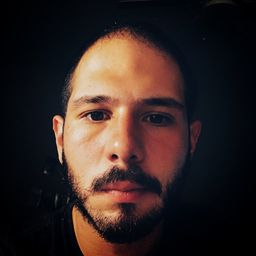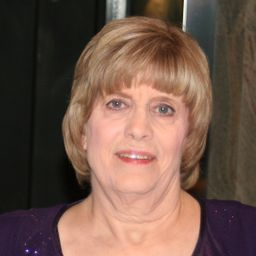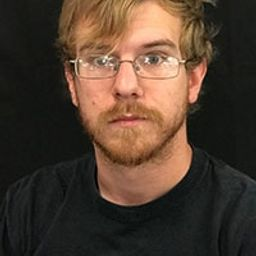💬 👋 We’re always here. Join our Discord to connect with other students 24/7, any time, night or day.Join Here!

## EducatorsAM+ 53 more educators

### Problem 1

Explain why the natural logarithmic function $y = \ln x$ is used much more frequently in calculus that the other logarithmic functions $y = \log_b x.$Carson M.

### Problem 2

Differentiate the function.

$f(x) = x \ln x - x$Carson M.

### Problem 3

Differentiate the function.

$f(x) = \sin (\ln x)$Doruk I.

### Problem 4

Differentiate the function.

$f(x) = \ln (\sin^2 x)$

fk
Fallo K.

### Problem 5

Differentiate the function.

$f(x) = \ln \frac {l}{x}$Carson M.

### Problem 6

Differentiate the function.

$y = \frac{l}{\ln x}$Doruk I.

### Problem 7

Differentiate the function.

$f(x) = \log_{10} (1 + \cos x)$Stephanie C.

### Problem 8

Differentiate the function.

$f(x) = \log_10 \sqrt x$Carson M.

### Problem 9

Differentiate the function.

$g(x) = \ln (xe^{-2x})$Carson M.

### Problem 10

Differentiate the function.

$g(t) = \sqrt {1 + \ln t}$Doruk I.

### Problem 11

Differentiate the function.

$F(t) = (\ln t)^2 \sin t$Carson M.

### Problem 12

Differentiate the function.

$h(x) = \ln (x + \sqrt {x^2 - 1})$

DV
Danie V.

### Problem 13

Differentiate the function.

$G(y) = \ln \frac {(2y + 1)^5}{\sqrt {y^2 + 1}}$Doruk I.

### Problem 14

Differentiate the function.

$P(v) = \frac {\ln v}{l - v}$Doruk I.

### Problem 15

Differentiate the function.

$F(s) = \ln \ln s$Doruk I.

### Problem 16

Differentiate the function.

$y = \ln \mid 1+ t - t^3 \mid$Doruk I.

### Problem 17

Differentiate the function.

$T(z) = 2^z \log_2 z$Carson M.

### Problem 18

Differentiate the function.

$y = \ln (\csc x - \cot x)$### Problem 19

Differentiate the function.

$y = \ln (e^{-x} + xe^{-x})$Doruk I.

### Problem 20

Differentiate the function.

$H(z) = \ln \sqrt {\frac {a^2 - z^2}{a^2 + z^2}}$Doruk I.

### Problem 21

Differentiate the function.

$y = \tan [\ln (ax + b)]$Doruk I.

### Problem 22

Differentiate the function.

$y = \log_2 (x \log_5 x)$Carson M.

### Problem 23

Find $y^{\prime}$ and $y^{\prime \prime}$
$y=\sqrt{x} \ln x$Carson M.

### Problem 24

Find $y'$ and $y".$

$y = \frac {\ln x}{l + \ln x}$Doruk I.

### Problem 25

Find $y'$ and $y".$

$y = \ln \mid \sec x \mid$Carson M.

### Problem 26

Find $y'$ and $y".$

$y = \ln (l + \ln x)$### Problem 27

Differentiate $f$ and find the domain of $f.$

$f(x) = \frac {x}{1 - \ln (x - 1)}$Doruk I.

### Problem 28

Differentiate $f$ and find the domain of $f.$

$f(x) = \sqrt {2 + \ln x}$Doruk I.

### Problem 29

Differentiate $f$ and find the domain of $f.$

$f(x) = \ln (x^2 - 2x)$Doruk I.

### Problem 30

Differentiate $f$ and find the domain of $f.$

$f(x) = \ln \ln \ln x$Doruk I.

### Problem 31

If $f(x) = \ln (x + \ln x),$ find $f'(1).$Doruk I.

### Problem 32

If $f(x) = \cos (\ln x^2),$ find $f'(1).$Doruk I.

### Problem 33

Find an equation of the tangent line to the curve at the given point.

$y = \ln (x^2 - 3x + 1), (3, 0)$### Problem 34

Find an equation of the tangent line to the curve at the given point.

$y = x^2 \ln x, (1, 0)$### Problem 35

If $f(x) = \sin x + \ln x,$ find $f'(x).$ Check that your answer is reasonable by comparing the graphs of $f$ and $f'.$Doruk I.

### Problem 36

Find equations of the tangent lines to the curve $y = (\ln x)/x$ at the points (1, 0) and $(e, 1/e).$ Illustrate by graphing the curve and its tangent lines.Doruk I.

### Problem 37

Let $f(x) = cx + \ln(\cos x).$ For what value of $c$ is $f'(\pi/4) = 6?$Doruk I.

### Problem 38

Let $f(x) = \log_b (3x^2 - 2).$ For what value of $b$ is $f'(1) = 3?$Doruk I.

### Problem 39

Use logarithmic differentiation to find the derivative of the function.

$y = (x^2 + 2)^2 (x^4 + 4)^4$Doruk I.

### Problem 40

Use logarithmic differentiation to find the derivative of the function.

$y = \frac {e^{-x} \cos^2 x}{x^2 + x + 1}$Doruk I.

### Problem 41

Use logarithmic differentiation to find the derivative of the function.

$y = \sqrt {\frac {x -1}{x^4 + 1}}$Doruk I.

### Problem 42

Use logarithmic differentiation to find the derivative of the function.

$y = \sqrt x e^{x^2 -x}(x + 1)^{2/3}$Bobby B.
University of North Texas

### Problem 43

Use logarithmic differentiation to find the derivative of the function.

$y = x^x$Carson M.

### Problem 44

Use logarithmic differentiation to find the derivative of the function.

$y = x^{\cos x}$Carson M.

### Problem 45

Use logarithmic differentiation to find the derivative of the function.

$y = x^{\sin x}$Carson M.

### Problem 46

Use logarithmic differentiation to find the derivative of the function.

$y = (\sqrt x)^x$### Problem 47

Use logarithmic differentiation to find the derivative of the function.

$y = (\cos x)^x$### Problem 48

Use logarithmic differentiation to find the derivative of the function.

$y = (\sin x)^{\ln x}$### Problem 49

Use logarithmic differentiation to find the derivative of the function.

$y = (\tan x)^{1/x}$### Problem 50

Use logarithmic differentiation to find the derivative of the function.

$y = (\ln x)^{\cos x}$Doruk I.

### Problem 51

Find $y'$ if $y$ = In $(x^2 + y^2).$Bobby B.
University of North Texas

### Problem 52

Find $y'$ if $x^y = y^x.$Bobby B.
University of North Texas

### Problem 53

Find a formula for $f^{(n)}(x)$ if $f(x) = \ln (x - 1).$Doruk I.

### Problem 54

Find $\frac {d^9}{dx^9}(x^8 \ln x).$Doruk I.

### Problem 55

Use the definition of derivative to prove that

$\displaystyle \lim_{x \to 0} \frac {\ln (1 + x)}{x} = 1$Doruk I.
Show that $\displaystyle \lim_{n \to x} (1 + \frac {x}{n})^n = e^x$ for any $x > 0.$